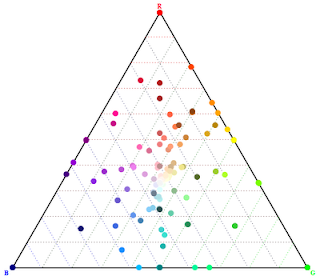## Dec 11, 2008

### How to make a Ternary Plot

For the reader who are curious about the post on Ternary PlotThe code is very simple, here is the mathematica notebook, and the pdf version if the notebook somehow doesn't work.

Sorry for the late reply, I seldom check the comments for the older posts.Anonymous said...

Thank you! I couldn't find another way to contact you, and was hoping you'd eventually read the old comments...Anonymous said...

A slightly simplified version:

Clear[a]
Clear[b]
Clear[c]
Clear[x]
Clear[y]
Clear[sum]

(*Expressing three parameters a,b, and c in terms of the Cartesian \
coordinates x and y with the constraint that a+b+c=sum*)

sum := 1

(*a, b, and c are defined so that 100% a corresponds to the lower \
left corner, 100% b to the lower right corner, and 100% c to the top \
corner.*)

b[x_, y_] := (x - y*Tan[Pi/6])*Cos[Pi/6]

a[x_, y_] := ((sum - x) - y*Tan[Pi/6])*Cos[Pi/6]

c[x_, y_] := y

(*Defining a function f (a,b,c)*)

f[x_, y_] := Cos[a[x, y]] + Sin[b[x, y]] + Sin[c[x, y]]

(*Drawing the contour plot*)

cp = ContourPlot[f[x, y], {x, 0, sum}, {y, 0, sum}]

(*Drawing the Triangle which bounds all allowed values of (a,b,c)*)

tr = Graphics[{Thick,
Line[{{0, 0}, {sum, 0}, {0.5*sum, Sqrt*sum/2}, {0, 0}}]},
ImageSize -> 300]

(*Putting it all together*)

Show[cp, tr]

Paul said...

First of all, this is fantastic. I use triangle plots all the time and and a new user of Mathematica, I was very happy to find this. And just a quick question, how do you think this would go plotting lines?

Tina said...

This is awesome!